# Minimum number of Jumps to reach End

Key takeaway: An excellent problem to learn time and space complexity optimization in a dynamic programming problem. The effcient single loop solution is intuitive and worth exploring.

### Let’s understand the problem

An array of positive integers is given, write a program to reach the last index using the minimum number of jumps.

• We are initially positioned at the first index of the array.
• Each element in the array represents the maximum jump length from that position. For example, if A[i] = x, this means that from index i we can jump to any one of the index i+1, i+2 … i+x.
• Assume that we can always reach the last index.
• There can be several ways to reach the end using the minimum number of jumps. So here our aim is to just return the minimum number of jump count.

Example 1

Input: A[] = [1, 3, 5, 8, 10, 2, 6, 7, 6, 8, 9], Output: 3

Explanation: 1-> 3 -> 8 -> 9 or 1-> 3 -> 10 -> 9

• Here we have 11 elements in the array, so start index = 0, end index = 10
• A = 1, so we can jump from the 0th index to the 1st index.
• Now A = 3, so we can jump max 3 steps from index 1. In other words, we can reach indices 2, 3, and 4 from the 1st index.
• A = 5. We can not reach the last index from index 2 because max reachable index from index 2 would be 2 + 5 = 7.
• A = 8. We can easily reach the last index from index 3 because the max reachable index from index 3 would be 3 + 8 = 11. In other words, we can take 7 step jump from index 3 to reach the last index 10.
• A = 10. We can easily reach the last index from index 4 because the max reachable index from index 4 would be 4 + 10 = 10. In other words, we can take 6 step jump from index 4 to reach the last index 10.

Example 2

Input: A[] = [2, 3, 1, 1, 4], Output: 2

Explanation: 2->3->4. The minimum number of jumps to reach the last index is 2. We jump 1 step from index 0 to 1, then 3 steps to the last index.

### Discussed solution approaches

• A brute force approach  using recursion
• A bottom-up approach using dynamic programming
• An efficient in-place approach  using a single loop

### A brute force approach  using recursion

Solution Idea

There can be many possible ways to reach the end, but we need to find a way using a minimum number of jumps. So the basic solution would be to calculate the jump count all possible ways to reach the end and return the minimum among those values. The critical question would be: How do we explore all possible ways to reach the end? Let's think!

When there is a requirement to explore all possible ways, we can think to apply the idea of recursion i.e. solution of the larger problem using the solution of smaller subproblems. Here is an intuition for the recursive solution: we start from the 0th index and recursively call for all the indices reachable from the 0th index. In other words, the minimum number of jumps to reach the last index can be calculated using the minimum number of jumps needed to reach the last index from the index reachable from the 0th index. (Think!)

Recursive Structure

• Suppose our initial function call is minJumps (A[], start, end), where end indices of the array A[] are start and end. So from the first index start, we can take a maximum single jump of i number of steps where 1 <= i <= A[start].
• What would be the smaller subproblem if we take a single jump of i number of steps from the start index? The answer would be simple: find the minimum number of jumps to reach the last index from (start + i)th index i.e. minJumps (A[], start + i, end).
• Now, for finding the minimum jump count, we recursively calculate jump count for all possible values of i (1 <= i <= A[start]) and find the minimum for all values of i. We also add 1 because we taking one jump to reach index i from the start index (Think!).

For all i reachable from start i.e. i = 1 to A[start]:

minJumps (A[], start, end) = 1 + min (minJump (A[], start + i, end ))

Base Case

This is a case of a single or zero element array where no jump is required to reach the end. i.e. if(start >= end), we return 0.

Solution Pseudocode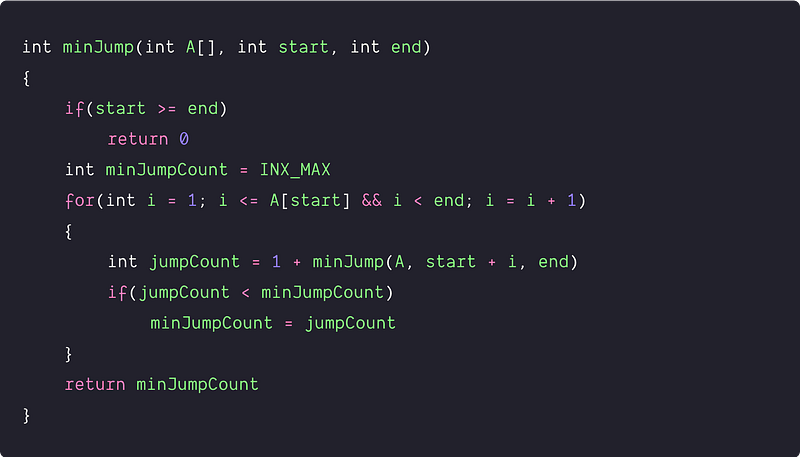Time and space complexity analysis

We can think from a simple perspective to calculate all possible ways to reach the end. There are two possibilities for each index from 1 to n-1: either we take a jump from that index or do not take a jump. So total number of possible ways = 2^(n-1), which is exponential time. Think! We can also think to analyze it by writing a recurrence relation.

• The time complexity to reach the end in n size array = T(n)
• After taking i number of the jump in the start, input size of the smaller sub-problem = n - i. So time complexity of the input size n - i = T(n - i)
• We are recursively calling for all the indexes reachable from the first index. So max possible jump count is equal to the value of A[start]. In the worst case, the max value of A[start] can be n - 1. (Think!).
• So here is the recurrence relation: T(n) = c + ∑(i = 1 to A[start]) T(n - i). If we put A[start] = n - 1, then we get the uppar bound: T(n) <= c + ∑(i = 1 to n - 1) T(n - i)
• We can also write in other way, if we put k = n - i: T(n) <= c + ∑(k = 1 to n - 1) T(k)
• If we solve the above recurrence using the recursion tree method, then the tree of recursive calls has 2^n - 1 leaf. (Think!). Time complexity = T(n) = O(2^n)

So finding the min jump is the exponential function of n, which is inefficient. In retrospect, this is not surprising because we are exploring all the possibilities. If we create a recursion tree for the above approach, we can clearly notice overlapping subproblems.

For example, if we observe the following picture, the sub-problem minJump(2, 5) is coming two times, minJump(3, 5) is coming 3 times, and so on.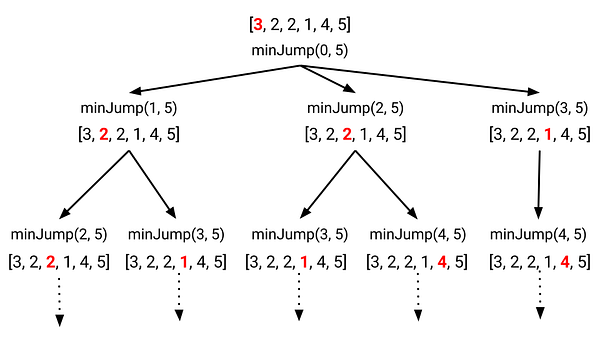Critical points to notice

• This is an optimization problem where solution space is exponentially large, but only a few solutions will provide the optimal solution.
• Sub-problems are overlapping i.e. we are solving the same subproblems again and again.
• In the worst case, the total number of different subproblems is equal to n i.e. sub-problems of size 1, sub-problem of size 2…up to sub-problem of size n. Our time complexity is exponential time because these n sub-problems will be repeated during the recursion (Think!)

### A bottom-up approach using dynamic programming

Solution Idea

Since we have identified that this is a dynamic programming problem, we can efficiently solve it using the bottom-up approach. Our aim here is to calculate the solution of the smaller sub-problems in an iterative way and store their result in a table.

• Table structure and size: There is only one variable that is decreasing by 1 during the recursion, so we can take a one-dimensional table jumps[n] to store the solutions of the n different sub-problems (The size of the table is equal to the total number of sub-problems). Here jump[i] indicates the minimum number of jumps needed to reach A[i] from A.
• Table initialization: We initialize the table by using the basic solution i.e. jumps = 0. When there is a single element in the array, there is no need to jump. We also initialize the remaining position of the jump[] array with the INT_MAX.
• Iterative structure to fill the table: We can define the iterative structure to fill the table by using the idea of the recursive solution. This approach of storing results in a table is similar to the longest increasing subsequence problem.

jumps[i] = min (jumps [j] + 1), For all j < i and i < j + A[j])

• Termination and returning final solution: Finally, our final answer will be stored at jumps[n-1], and we return this value.

Solution Pseudocode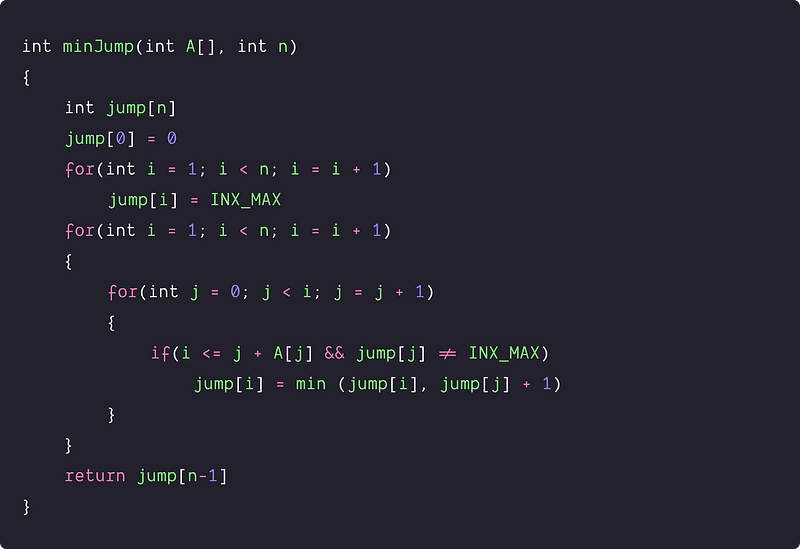Time and space complexity analysis

Time complexity = Time complexity of initializing jump[] array + Time complexity of nested loops to store values in jump[] array = O(n) + O(n^2) = O(n^2). Similarly, space complexity = O(n) for using n size jump[] array.

### An efficient in-place approach  using a single loop

Solution Idea

Now the dynamic programming solution is much efficient than a brute force solution. But critical questions are: can we further improve the time complexity or space complexity, or both? Can we try to remove the inner loop of the above solution? Can we track the value of the minimum jump using some variable? Here is an improvement insight:

From any jump point i, we can reach any index from (i + 1) to (i + A[i]), and there will be some value between range A [i + 1] to A [i + A[i]], which can provide the farthest reach from that range. So we define the lower and upper end of the current jump points and calculate the farthest reach in that range.

Whenever we reach the upper endpoint of the range, we update the upper end with the value of the farthest reach and increment the jump count. We continue this process till the value of the farthest reachable index is greater than n - 1.

Let's define four variables to simulate the above process:

• jump: To store the value of jump count. Initially jump = 0.
• currStart and currEnd: To store the range of the current jump point. Initially currStart = 1, currEnd = A.
• currMaxReach: To store the farthest reach possible in the range [currStart, currEnd]. Initially currMaxReach = A.

Solution Steps

• We initialize jump = 0, currStart = 1, currEnd = A, currMaxReach = A.
• Now we traverse the array from currStart = 1 to n-2 and continue updating the currMaxReach. Here currStart will be less than n-1 because when we reach the last one, we don't need to jump again.
• Once currStart == currEnd, we trigger jump and update the range of the next jump point. To update the range, we update the value of currEnd with curMaxReach.
• During the update of currMaxReach, we also need to check If(currMaxReach > n-1). If it is true, we can directly reach the end of the array from the currStart by taking a single jump. So we increment the value of the jump and break the loop.
• By the end of the loop, we return the value stored in the jump.

Solution Pseudocode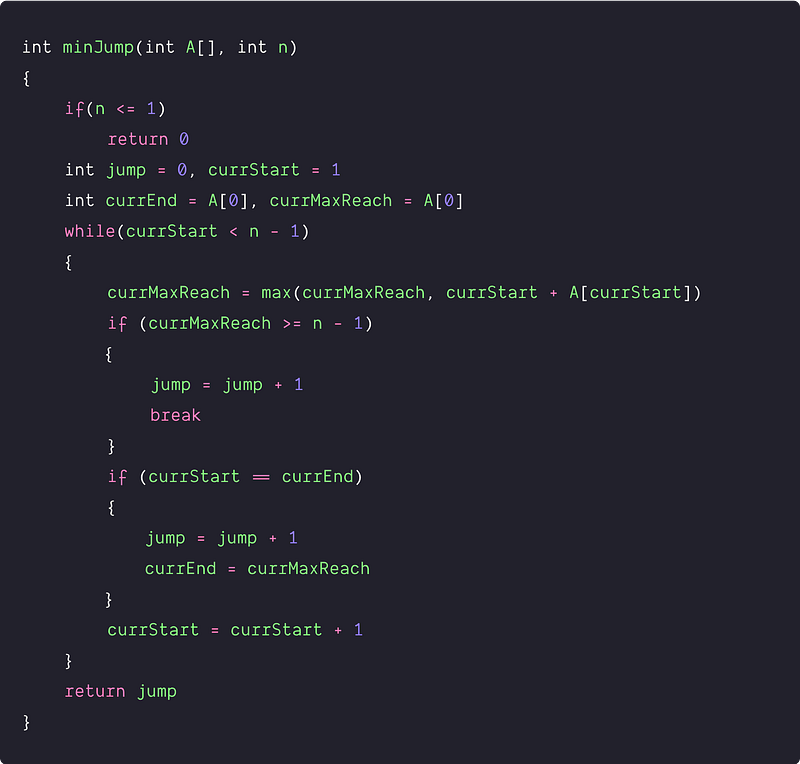Another implementation idea

• In this approach, we define three variables: currMaxReachstepsCount, and jump. We define both jump and stepsCount variable to the value of the first index of the array. Here currMaxReach is the maximum we can reach from that index, which is the index plus the value of the index (the jump value). So, we keep updating it in each iteration so that whenever we move forward, the variable stores the max reach by using currMaxReach = max(currMaxReach, A[i]+i)
• Also, at each iteration, we reduce our stepsCount variable by 1. As we move forward, we consume 1 step each time. So, whenever we run out of steps, it means we need to take one jump. So, we increase the jump variable and update the stepsCount variable to the value (currMaxReach - i) i.e. the maximum reach possible from the current index. So, we can take those steps, and then we need to jump again.
• So, we return jump+1 as our output in this solution since we only jump after running out of steps. Also, we need to note that: we moved only till the second last element and not the last element since at the last step, we do not need to consume one more step as we are already there and no need to jump more.

Solution Pseudocode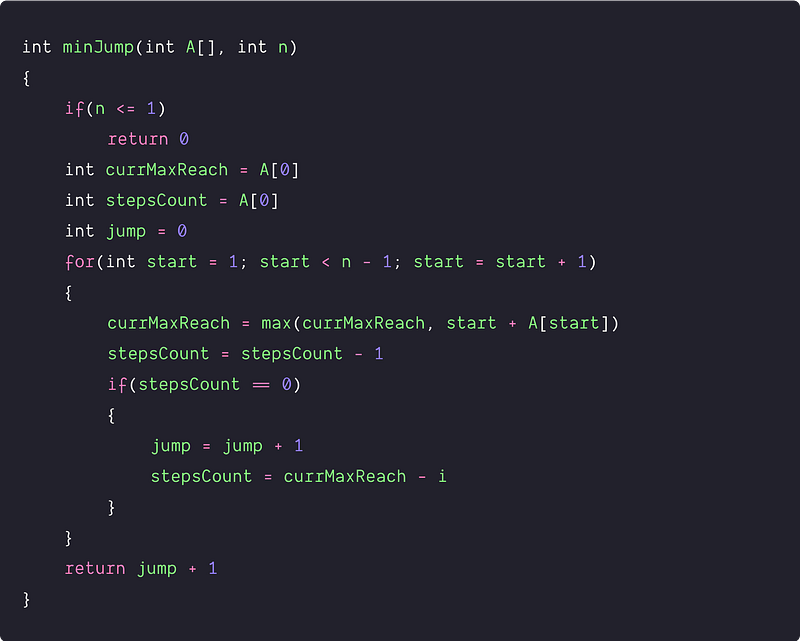Time and space complexity analysis

In both the single loop solution, we are doing constant operations at each iteration. So time complexity = O(n). We are using constant extra space, so space complexity = O(1)

### Critical ideas to think!

• Can we think of implementing the single loop solution by traversing the array from right to left instead of left to right?
• How would we modify the above approach if there is no guarantee to reach the end? In other words, return true if we can reach the last index, or false otherwise.
• Does the single solution look like a greedy approach?
• What would be the space complexity of the recursive solution?
• How do we modify the above approaches to output the elements involved in reaching the end-point using the minimum number of jumps?

### Suggested coding problems to practice

Enjoy learning, Enjoy problem-solving, Enjoy algorithms!

Subscribe to get well-designed content on data structures and algorithms, machine learning, system design, oops, and mathematics. enjoy learning!

### We Welcome Doubts and Feedback!